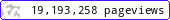•••••MadAsMaths.com :: I.Y.G.B. Practice Papers :: Special & Extension Papers :: SP Practice Papers

I.Y.G.B. SP PRACTICE PAPERS

These Special Papers follow the Common Core Syllabus, C1 to C4, introduced in 2005.
The aim of these papers is to give extremely able students the very best in difficulty.

Note that the solutions to these papers are available on request, however a maximum of 3 sets of solutions can be provided per request, and no repeated requests.

 sp_a.pdfsp_a_solutions.pdfsp_b.pdfsp_b_solutions.pdfsp_c.pdfsp_c_solutions.pdfsp_d.pdfsp_d_solutions.pdfsp_e.pdfsp_e_solutions.pdfsp_f.pdfsp_f_solutions.pdfsp_g.pdfsp_g_solutions.pdfsp_h.pdfsp_h_solutions.pdfsp_i.pdfsp_i_solutions.pdfsp_j.pdfsp_j_solutions.pdfsp_k.pdfsp_k_solutions.pdfsp_l.pdfsp_l_solutions.pdfsp_m.pdfsp_m_solutions.pdfsp_n.pdfsp_n_solutions.pdfsp_o.pdfsp_o_solutions.pdfsp_p.pdfsp_p_solutions.pdfsp_q.pdfsp_q_solutions.pdfsp_r.pdfsp_r_solutions.pdfsp_s.pdfsp_s_solutions.pdfsp_t.pdfsp_t_solutions.pdfsp_u.pdfsp_u_solutions.pdfsp_v.pdfsp_v_solutions.pdfsp_w.pdfsp_w_solutions.pdfsp_x.pdfsp_x_solutions.pdfsp_y.pdfsp_y_solutions.pdfsp_z.pdfsp_z_solutions.pdf